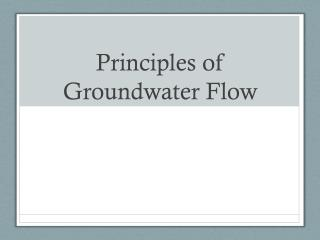# Principles of Groundwater Flow - PowerPoint PPT PresentationDownload PresentationPrinciples of Groundwater Flow

Principles of Groundwater FlowDownload Presentation## Principles of Groundwater Flow

- - - - - - - - - - - - - - - - - - - - - - - - - - - E N D - - - - - - - - - - - - - - - - - - - - - - - - - - -

1. ### Principles of Groundwater Flow

2. Hydraulic Head Bernoulli’s Equation Hydrostatic Pressure
3. Hydraulic Head Bernoulli’s Equation Hydrostatic Pressure
4. Hydraulic Head Total Hydraulic Head (drop velocity – why?) Or Force Potential (driver) Bernoulli’s equation suggests this should be constant, but clearly it is not – what’s going on?
5. How to measure it? Piezometer
6. Validity and Applicability of Darcy’s Law Reynolds Number Re = (rvd/m) r– density v – velocity d – characteristic distance m- viscosity
7. Equations of Flow – Confined Aquifers Combine Darcy’s Law and Conservation of Mass (Derivation on Pages 126-128 of Fetter) Governing Equation Transmissivity (average over z direction)
8. Unconfined Aquifers Without a confining upper layer the governing equation changes a little Boussinesq Equation What makes this equation so difficult to deal with?
9. Direction of Groundwater Flow Potential and Equipotential Lines What direction is the flow in
10. Steady Flow in Confined Aquifer
11. Steady Flow in Confined Aquifer
12. Steady Flow in an Unconfined A quifer
13. Steady Flow in an Unconfined A quifer
14. Groundwater Divides Can our equations predict these? Source or sink
15. Groundwater Divides Can our equations predict these?
16. Groundwater Divides If h=h1 at x=0 and h =h2 at x=L
17. Groundwater Divides Discharge per unit width
18. Groundwater Divides Location of GW Divide is where q’=0